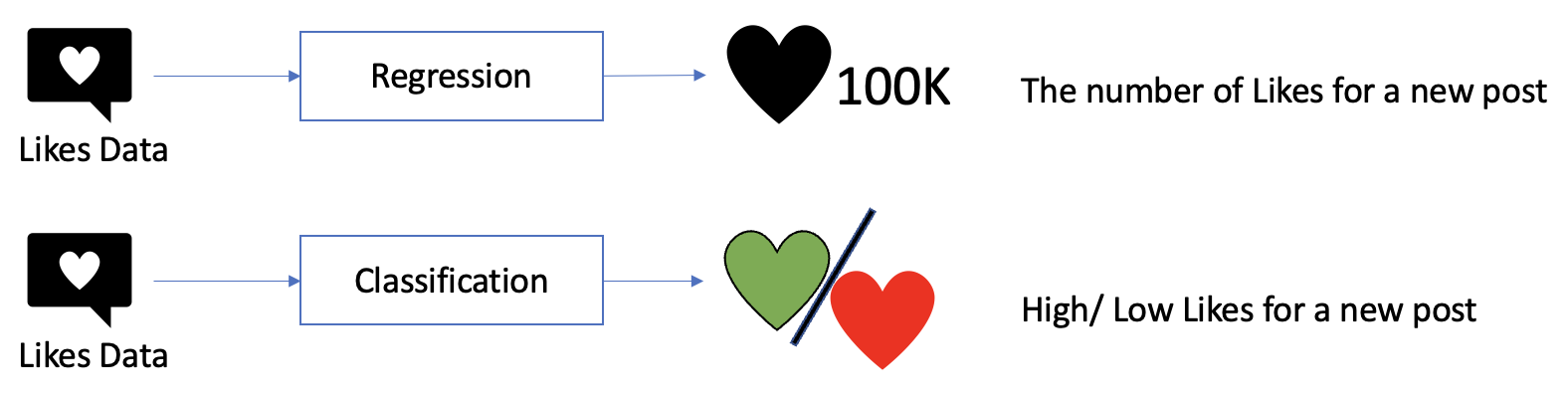# Comparing the Results: Classification vs. Regression Models in Machine LearningWhen analyzing data, one may come across two main types of machine learning models: classification and regression. Both models are used to predict outcomes based on input variables, but they differ in their objectives and output formats. In this blog post, we will compare the classification and regression results to understand how they differ in their predictions and applications.

Classification models are used when the outcome variable is categorical or binary, meaning it has a limited number of options. The objective of a classification model is to assign new observations to one of the predefined categories based on the input variables. Examples of classification problems include spam detection, image recognition, and sentiment analysis. The output of a classification model is a probability or score indicating the likelihood of a new observation belonging to each category.

On the other hand, regression models are used when the outcome variable is continuous or numeric, meaning it can take any value within a certain range. The objective of a regression model is to predict the value of the outcome variable based on the input variables. Examples of regression problems include stock price prediction, sales forecasting, and weather forecasting. The output of a regression model is a numerical value indicating the predicted outcome variable.

To evaluate the performance of classification and regression models, we can use different evaluation metrics depending on the problem and the model’s objective. In classification models, we can use metrics such as accuracy, precision, recall, F1-score, and ROC-AUC to evaluate the performance of the model. In regression models, we can use metrics such as mean squared error (MSE), root mean squared error (RMSE), and R-squared to evaluate the performance of the model.

One example of a problem that can be solved using both regression and classification models is predicting the number of likes on a tweet. The regression model can be used to predict the exact number of likes a tweet will receive based on input variables such as the length of the tweet, the use of certain keywords or hashtags, and the time of day it was posted. The classification model, on the other hand, can be used to categorize tweets into high or low engagement categories based on the number of likes they are likely to receive. This can be useful for social media marketers who want to quickly identify the most engaging tweets and focus their efforts on those tweets that are likely to receive a high number of likes. The regression model, however, can provide more precise predictions for specific tweets that are tailored to a particular audience or marketing campaign.

However, there is no one common evaluation metric to compare the results of a classification model with a regression model! So, there is no straightforward way!

One non-straightforward way to compare the results of a binary classification model with a regression model is to convert the regression continuous target values into binary by finding an optimal threshold. But, first, you need to make sure that both models resolve the same problem and are trained using the same dataset. Second, to achieve maximum accuracy, you need to determine the optimal threshold for the regression model. An optimal threshold should separate the testing records into categories of 0 and 1 classes. After that, you need to convert the regression model’s target values into binary. Finally, you now can compare the labeled regression results with the binary classification model results using the F1-score.

Aldous, K, An, J, and Jansen, B. J. (2022) What Really Matters?: Characterizing and Predicting User Engagement of News Postings Using Multiple Platforms, Sentiments, and TopicsBehaviour & Information Technologyhttps://doi.org/10.1080/0144929X.2022.2030798​